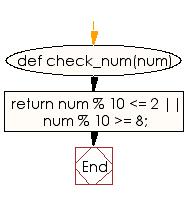﻿ Ruby Basic exercises: Check a given non-negative number and return true if num is within 2 of a multiple of 10 - w3resource# Ruby Basic Exercises: Check a given non-negative number and return true if num is within 2 of a multiple of 10

## Ruby Basic: Exercise-46 with Solution

Write a Ruby program to check a given non-negative number and return true if num is within 2 of a multiple of 10.

Ruby Code:

``````def check_num(num)
return num % 10 <= 2 || num % 10 >= 8;
end
print check_num(9),"\n"
print check_num(13),"\n"
print check_num(22),"\n"
print check_num(-12),"\n"
print check_num(0)
``````

Output:

```true
false
true
true
true
```

Flowchart:Ruby Code Editor: